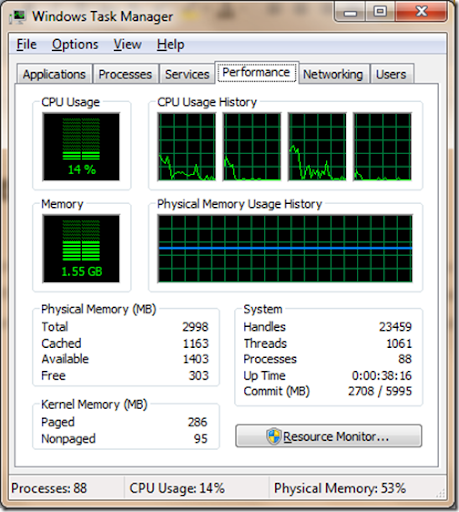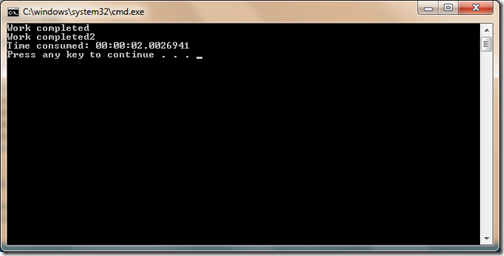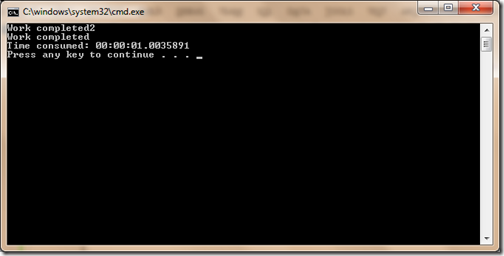Sunday, December 16, 2012

## Parallel task in C# 4.0

In today’s computing world  is all about Parallel processing. You have multicore CPU where you have different core doing different work parallel or its doing same task parallel. For example I am having 4-core CPU as follows. So the code that I write should take care of this. C# does provide that kind of facility to write code for multi core CPU with task parallel library. We will explore that in this post.Now Let’s write a program that will have some will have some CPU intensive code that will take some time to complete work.  First we will use normal way of calling this task and measure the time and then we will use Task Parallel library feature of C# 4.0. Following is a code for that.

```using System;
using System.Diagnostics;

{
class Program
{
static void Main(string[] args)
{
Stopwatch stopWatch = new Stopwatch();
stopWatch.Start();
DoSomeWork();
DoSomework2();
stopWatch.Stop();
Console.WriteLine("Time consumed: {0}", stopWatch.Elapsed);
}

public static void DoSomeWork()
{
Console.WriteLine("Work completed");
}
public static void DoSomework2()
{
Console.WriteLine("Work completed2");
}

}
}
```

As you can see in above code that we have two cpu intensive method DoSomeWork and DoSomeWork2 and we have called it one by one. Now let’s run the console application.Here you can see that it has taken almost 2 seconds to complete this task. To use parallel task library we have to use System.Threading.Tasks name space. So I have changed the above code like following to get advantage of Parallel Task.

```using System;
using System.Diagnostics;

{
class Program
{
static void Main(string[] args)
{
Stopwatch stopWatch = new Stopwatch();
stopWatch.Start();
Parallel.Invoke(
new Action(DoSomeWork),
new Action(DoSomework2)
);
stopWatch.Stop();
Console.WriteLine("Time consumed: {0}", stopWatch.Elapsed);
}

public static void DoSomeWork()
{
Console.WriteLine("Work completed");
}
public static void DoSomework2()
{
Console.WriteLine("Work completed2");
}

}
}
```

So here you can see I have invoked two CPU consuming task parallel invoke and used action delegate to call that. Now let’s run that and see how much time it will take.Here you can see it has taken 1 second to complete same task as it has completed this tasks parallel. Hope you like it. Stay tuned for more.Share: